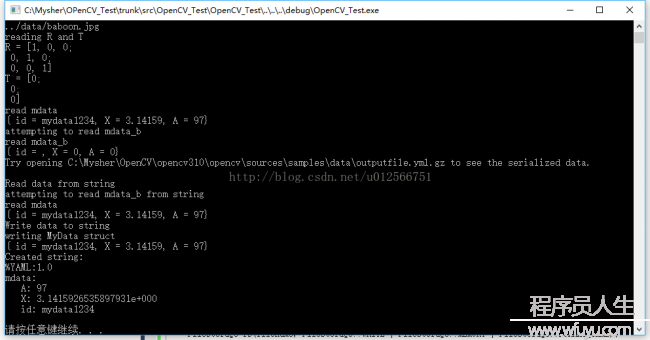# OpenCV3.0 Examples学习笔记（8）-filestorage.cpp

Example运行截图
Example分析
Example代码

FileStorage类提供了对xml，yml文件的读写操作。
FileStorage 具体定义可以参见cv::FileStorage Class Reference

Example截图%YAML:1.0 images:    - "image1.jpg"    - "myfi.png"    - "../data/baboon.jpg" R: !!opencv-matrix    rows: 3    cols: 3    dt: d    data: [ 1., 0., 0., 0., 1., 0., 0., 0., 1. ] T: !!opencv-matrix    rows: 3    cols: 1    dt: d    data: [ 0., 0., 0. ] mdata:    A: 97    X: 3.1415926535897931e+000    id: mydata1234

Example分析
1.使用FileStorage 写文件
1.1.打开1个文件并准备进行写操作
FileStorage fs(filename, FileStorage::WRITE);

（1）FileStorage处理支持xml，yml外， 还支持gz的紧缩格式。

1.2.创建images结点，并写入"image1.jpg"， "myfi.png" ，"../data/baboon.jpg"3个字段
fs << "images" << "[";

fs << "image1.jpg" << "myfi.png" << "../data/baboon.jpg";
cout << "image1.jpg" << " myfi.png" << " ../data/baboon.jpg" << endl;

fs << "]";

1.3.在文件中嵌入Mat数据，并分别生成节点R，T
cout << "writing mats\n";
Mat R =Mat_<double>::eye(3, 3),T = Mat_<double>::zeros(3, 1);
cout << "R = " << R << "\n";
cout << "T = " << T << "\n";
fs << "R" << R;
fs << "T" << T;

1.4.在文件中嵌入自定义数据，并生成mdata节点
cout << "writing MyData struct\n";
MyData m(1);
fs << "mdata" << m;
cout << m << endl;

2.使用FileStorage 读取文件
2.1.打开1个文件并准备进行读操作

if (!fs.isOpened())
{
cerr << "failed to open " << filename << endl;
help(av);
return 1;
}

2.2.读取文件中images节点
FileNode n = fs["images"];
if (n.type() != FileNode::SEQ)
{
cerr << "images is not a sequence! FAIL" << endl;
return 1;
}

2.3.遍历images节点中字符串
FileNodeIterator it = n.begin(), it_end = n.end();
for (; it != it_end; ++it)
{
cout << (string)*it << "\n";
}

2.4.读取R，T节点（依照Mat）
Mat R, T;
cout << "reading R and T" << endl;

fs["R"] >> R;
fs["T"] >> T;

cout << "R = " << R << "\n";
cout << "T = " << T << endl;

2.5.读取mdata节点（依照自定义结构体MyData
MyData m;
fs["mdata"] >> m;

cout << m << endl;

2.6.尝试读取不存在的节点，验证鲁棒性
cout << "attempting to read mdata_b\n";   //Show default behavior for empty matrix
fs["mdata_b"] >> m;
cout << m << endl;

3.从string中读
3.1创建string
cout << "Read data from string\n";
string dataString =
"%YAML:1.0\n"
"mdata:\n"
"   A: 97\n"
"   X: 3.1415926535897931e+00\n"
"   id: mydata1234\n";

3.2创建自定义结构体MyData 对象
MyData m;

3.3创建对内存读取的FileStorage 对象

（1）FileStorage::MEMORY，表示从source读数据，或向内部缓存写入数据（由FileStorage::release返回）

3.4将dataString内容写入，MyData 对象m中
cout << "attempting to read mdata_b from string\n";   //Show default behavior for empty matrix
fs["mdata"] >> m;
cout << m << endl;

4.向string中写数据
4.1打开1个文件并进行写操作
cout << "Write data to string\n";
FileStorage fs(filename, FileStorage::WRITE | FileStorage::MEMORY | FileStorage::FORMAT_YAML);

（1）由于包括FileStorage::MEMORY，所以其实其实不会写入文件，只是在内存中操作。

4.2创建自定义结构体MyData 对象m
cout << "writing MyData struct\n";
MyData m(1);

4.3将数据m写入mdata节点
fs << "mdata" << m;
cout << m << endl;

4.4关闭对象，并获得其内容
string createdString = fs.releaseAndGetString();
cout << "Created string:\n" << createdString << "\n";

Example源码

```/*
* filestorage_sample demonstrate the usage of the opencv serialization functionality
*/

#include "opencv2/core/core.hpp"
#include <iostream>
#include <string>

using std::string;
using std::cout;
using std::endl;
using std::cerr;
using std::ostream;
using namespace cv;

static void help(char** av)
{
cout << "\nfilestorage_sample demonstrate the usage of the opencv serialization functionality.\n"
<< "usage:\n"
<<  av << " outputfile.yml.gz\n"
<< "\n   outputfile above can have many different extenstions, see below."
<< "\nThis program demonstrates the use of FileStorage for serialization, that is use << and >>  in OpenCV\n"
<< "For example, how to create a class and have it serialize, but also how to use it to read and write matrices.\n"
<< "FileStorage allows you to serialize to various formats specified by the file end type."
<< "\nYou should try using different file extensions.(e.g. yaml yml xml xml.gz yaml.gz etc...)\n" << endl;
}

struct MyData
{
MyData() :
A(0), X(0), id()
{
}
explicit MyData(int) :
A(97), X(CV_PI), id("mydata1234")
{
}
int A;
double X;
string id;
void write(FileStorage& fs) const //Write serialization for this class
{
fs << "{" << "A" << A << "X" << X << "id" << id << "}";
}
{

A = (int)node["A"];
X = (double)node["X"];
id = (string)node["id"];
}
};

//These write and read functions must exist as per the inline functions in operations.hpp
static void write(FileStorage& fs, const std::string&, const MyData& x){
x.write(fs);
}
static void read(const FileNode& node, MyData& x, const MyData& default_value = MyData()){
if(node.empty())
x = default_value;
else
}

static ostream& operator<<(ostream& out, const MyData& m){
out << "{ id = " << m.id << ", ";
out << "X = " << m.X << ", ";
out << "A = " << m.A << "}";
return out;
}
int main(int ac, char** av)
{
if (ac != 2)
{
help(av);
return 1;
}

string filename = av;

//write
{
FileStorage fs(filename, FileStorage::WRITE);

cout << "writing images\n";
fs << "images" << "[";

fs << "image1.jpg" << "myfi.png" << "../data/baboon.jpg";
cout << "image1.jpg" << " myfi.png" << " ../data/baboon.jpg" << endl;

fs << "]";

cout << "writing mats\n";
Mat R =Mat_<double>::eye(3, 3),T = Mat_<double>::zeros(3, 1);
cout << "R = " << R << "\n";
cout << "T = " << T << "\n";
fs << "R" << R;
fs << "T" << T;

cout << "writing MyData struct\n";
MyData m(1);
fs << "mdata" << m;
cout << m << endl;
}

{

if (!fs.isOpened())
{
cerr << "failed to open " << filename << endl;
help(av);
return 1;
}

FileNode n = fs["images"];
if (n.type() != FileNode::SEQ)
{
cerr << "images is not a sequence! FAIL" << endl;
return 1;
}

FileNodeIterator it = n.begin(), it_end = n.end();
for (; it != it_end; ++it)
{
cout << (string)*it << "\n";
}

Mat R, T;
cout << "reading R and T" << endl;

fs["R"] >> R;
fs["T"] >> T;

cout << "R = " << R << "\n";
cout << "T = " << T << endl;

MyData m;
fs["mdata"] >> m;

cout << m << endl;

cout << "attempting to read mdata_b\n";   //Show default behavior for empty matrix
fs["mdata_b"] >> m;
cout << m << endl;

}

cout << "Try opening " << filename << " to see the serialized data." << endl << endl;

{
cout << "Read data from string\n";
string dataString =
"%YAML:1.0\n"
"mdata:\n"
"   A: 97\n"
"   X: 3.1415926535897931e+00\n"
"   id: mydata1234\n";
MyData m;
cout << "attempting to read mdata_b from string\n";   //Show default behavior for empty matrix
fs["mdata"] >> m;
cout << m << endl;
}

//write to string
{
cout << "Write data to string\n";
FileStorage fs(filename, FileStorage::WRITE | FileStorage::MEMORY | FileStorage::FORMAT_YAML);

cout << "writing MyData struct\n";
MyData m(1);
fs << "mdata" << m;
cout << m << endl;
string createdString = fs.releaseAndGetString();
cout << "Created string:\n" << createdString << "\n";
}

return 0;
}```

1.《OpenCV使用FileStorage保存Mat数据
2.《OpenCV编程->FileStorage解析
3.《OpenCV FileStorage 使用记录
4.《Yml文件的读取与写入
5.《OpenCV —FileStorage类的数据读写操作与示例
6.《OpenCV持久化（1）------分隔线----------------------------

------分隔线----------------------------0粉丝
2关注